https://doi.org/10.5194/amt-16-4961-2023
https://doi.org/10.5194/amt-16-4961-2023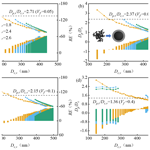# Numerical investigation on retrieval errors of mixing states of fractal black carbon aerosols using single-particle soot photometer based on Mie scattering and the effects on radiative forcing estimation

Jia Liu, Guangya Wang, Cancan Zhu, Donghui Zhou, and Lin Wang
Abstract

The mixing state of black carbon (BC) aerosols, which is the diameter ratio of coated particle to BC core (${D}_{\mathrm{p}}/{D}_{\mathrm{c}}$), can be retrieved by the single-particle soot photometer (SP2). However, the retrieved ${D}_{\mathrm{p}}/{D}_{\mathrm{c}}$ contains errors, because the core–shell model and Mie scattering calculation are normally employed in the retrieval principle of SP2 and the spherical core–shell structure seriously deviated from the real morphology of coated BC. In this study, fractal models are constructed to represent thinly and thickly coated BC particles for optical simulations, the differential scattering cross-sections are selected as references to conduct optical retrieval of particle diameter (Dp) based on Mie theory, just like the retrieval principle of SP2, and the volume equivalent diameter of BC core (Dc) is the same for fractal and spherical models. Then, the retrieval errors of the mixing state (${D}_{\mathrm{p}}/{D}_{\mathrm{c}}$) of BC are investigated from numerical aspects, and the estimation accuracy of BC radiative forcing is analyzed through the simple forcing efficiency (SFE) equation with SP2 retrieval results taken into consideration. Results show that SP2 retrieved ${D}_{\mathrm{p}}/{D}_{\mathrm{c}}$ based on Mie theory underestimates the realistic ${D}_{\mathrm{p}}/{D}_{\mathrm{c}}$ of coated BC at most particle sizes. The retrieval errors of ${D}_{\mathrm{p}}/{D}_{\mathrm{c}}$ of thinly coated BC for both single particles and particle groups are larger than those of thickly coated BC. In addition, evaluation errors of radiative forcing of coated BC caused by retrieval errors of SP2 are up to about 55 % and 95 % at 1064 and 532 nm, respectively. This study provides meaningful referential understandings of the retrieved ${D}_{\mathrm{p}}/{D}_{\mathrm{c}}$ of SP2 based on Mie scattering.

Share
Dates
1 Introduction

Black carbon (BC) produced from the incomplete combustion of biomass and fossil fuels is considered to be an important contributor to global warming (Zhang et al., 2021). Black carbon aerosols directly affect the climate by absorbing shortwave solar radiation to heat the atmosphere, and they also indirectly affect the climate through the complex interaction with clouds (Zhao et al., 2022). The bare BC just emitted from the source has poor hygroscopicity, making it difficult to form cloud condensation nuclei (CCN) (Zhuang et al., 2013). When the hydrophobic BC is mixed with hydrophilic aerosol during the aging process, the mixed BC aerosol also becomes hydrophilic, which further acts as CCN and participates in microphysical processes of clouds, thus affecting the effective radius and number density of cloud droplets, cloud volume and lifetime, and ultimately the climate (Ching et al., 2016). When deposited to the surface of snow and ice, BC further reduces the snow albedo and accelerates the melting process (Jacobson, 2004). In addition, BC aerosols also affect the air pollution process by altering the thermal structure of the planetary boundary layer, which further suppresses the vertical diffusion of air pollutants, enhances haze events, harms human health, and reduces atmospheric visibility (Huang et al., 2018).

The non-spherical fractal morphology of BC has attracted extensive attention. Freshly emitted black carbon particles are chain-like aggregates consisting of a large number of near-spherical monomers. During the aging process in the atmospheric environment, BC will be coated by other species, and their aggregate morphology tends to be more compact (China et al., 2013). Combined observation and simulation carried out by China et al. (2015) showed that Mie calculations provide reasonable approximations for compact soot above remote marine clouds, and the distinction of radiative forcing estimated using Mie theory and using discrete dipole approximation is within 12 % for a high surface albedo. The aging process causes variations in the mixing states of BC particles and results in significant changes in the optical parameters including absorption properties, which in turn affects the radiative forcing of BC and brings great uncertainties to the assessment of the climate effects of BC (Wu et al., 2018; Zeng et al., 2019). Therefore, the measurement and monitoring of the mixing states of BC particles have drawn much attention in the field of atmospheric aerosol observation. Currently, the mixing states of rBC-containing particles are mainly characterized using the following methods: the particle diameter ratio of the whole particles to the BC core (${D}_{\mathrm{p}}/{D}_{\mathrm{c}}$), the coating thickness (CT), the SP2 measured numerical fractions of thinly and thickly coated rBC, and the mass ratio of the coating material to the BC core (MR). Single-particle soot photometer (SP2) can effectively retrieve the mixing state (${D}_{\mathrm{p}}/{D}_{\mathrm{c}}$) of BC aerosol based on Mie scattering and has been widely used in field and laboratory observations. Liu et al. (2014) employed SP2 to measure the particle size and mixing state of BC aerosols in London during winter and analyzed their emission sources, and results showed that the ${D}_{\mathrm{p}}/{D}_{\mathrm{c}}$ of BC particles emitted from three types of sources in the west, southeast, and east were 1.28, 1.45 and 1.69, respectively. Liu et al. (2022) measured MR under different time backgrounds with the assistance of the tandem system of a Couette centrifugal particle mass analyzer (CPMA) and an SP2 and verified that the mixing state of BC was affected by both relative humidity (RH) and pollutant concentrations. Zhang et al. (2020) employed SP2 to characterize fresh BC particles emitted from four different emission sources and found that coatings are often already present in freshly emitted BC particles, and diesel vehicles emit BC particles with the least coatings (${D}_{\mathrm{p}}/{D}_{\mathrm{c}}$ 1.25), while crop residues emit BC particles with the most coatings (${D}_{\mathrm{p}}/{D}_{\mathrm{c}}$ 1.75). The application of SP2 has remarkably improved the understanding of the mixing state of freshly emitted and aged BC particles.

The SP2 measures the mass and differential scattering cross-section of each single BC particle based on the combination of laser-induced incandescent light technology and light scattering measurement technology. In the optical cavity, when a coated BC particle vertically passes through the high-energy laser beam at a wavelength of 1064 nm, there are two scattering signal detectors to collect the scattering signal over certain solid angles at forward and backward directions, respectively (Schwarz et al., 2006). Gao et al. (2007) developed the leading-edge-only (LEO) fit method to deal with the collected scattering signal for SP2 with a position-sensitive detector. The undisturbed leading edge of the scattering signal is employed to construct a Gaussian scattering function, and then the Gaussian function can be used to determine the differential scattering cross-section of the BC-containing particle. On the other hand, for SP2 without a position-sensitive detector, Moteki and Kondo (2008) proposed measurement of the time-dependent differential scattering cross-section (ΔCsca(t)) as each particle flowing across the Gaussian laser beam, so that the differential scattering cross-section for coated BC particles can be further obtained. In general, the coated particles rapidly absorb the laser energy, the coating is heated to vaporization at first, and then the refractory BC (rBC) is heated and emits incandescent light (Zhao et al., 2021). At 1064 nm wavelength, when the rBC with particle masses range between the lower and upper detection limits of SP2, the intensity of the incandescent light signal is proportional to the mass of rBC, and the volume equivalent particle diameter of rBC can be obtained based on the preset density (1.80 g cm−3) (Moteki and Kondo, 2010). Mie scattering theory, which assumes that the coated BC particle has a concentric core–shell structure consisting of coating sphere and BC sphere, is usually employed to retrieve the optical equivalent diameter of the coated BC based on differential scattering cross-section measured by SP2 (Schwarz et al., 2008; Kompalli et al., 2021). Finally, the particle size ratio of the whole particle to the BC core (${D}_{\mathrm{p}}/{D}_{\mathrm{c}}$) can be obtained. Experimental results obtained by Laborde et al. (2012) showed that Mie scattering theory can be employed to retrieve the coating thickness of aged BC particles based on SP2 measurements. However, comparisons conducted by Scarnato et al. (2013) revealed that the scattering and absorption of the internally mixed BC calculated by the discrete dipole approximation (DDA) and the Mie scattering theory may be considerably different at 1000 nm (close to the 1064 nm used by SP2). Moteki et al. (2014) emphasized that the optical properties simulated by Mie theory deviate from the SP2 observations by as much as 40 % and are affected by the total particle mass, the rBC mass, and the refractive index of oleic acid coating. Schwarz et al. (2015) also proposed that when the SP2 was used to quantify the water-uptake of BC particles coated by ammonium sulfate, the uncertainty of SP2 measured results was mainly caused by the significant deviations in predicting the SP2 scattering properties of the BC particles using Mie scattering. In summary, it can be deduced that there are unavoidable errors in the retrieved ${D}_{\mathrm{p}}/{D}_{\mathrm{c}}$ based on Mie theory, because the core–shell model used in the retrieval of optical equivalent particle size Dp does not conform to the non-spherical complex morphology of the coated BC particles. At present, the retrieval error in${D}_{\mathrm{p}}/{D}_{\mathrm{c}}$ of coated BC based on SP2 measurement results is difficult to quantify directly through experimental investigations. Nevertheless, the rapid developments of both morphology modeling and optical simulation of coated BC particles provide an investigative strategy for evaluating the retrieval accuracy of ${D}_{\mathrm{p}}/{D}_{\mathrm{c}}$.

Based on the microscopic morphology of coated BC, a series of models have been constructed in previous studies to investigate the optical properties of BC. Wu et al. (2014) constructed a fractal closed-cell model with concentric bilayer spheres as monomers and the optical properties at 450–1000 nm, calculated using the multiple-sphere T-matrix method (MSTM), and they found that the optical properties of the closed-cell model differ significantly from the calculated results of the core–shell model based on Mie scattering theory. Zeng et al. (2019) developed the coated-aggregate model and calculated the results using the MTSM, which showed that extinction of coated BC is significantly enhanced due to the increase in scattering and that the enhancement depends on both the amount and hydrophilicity of the coatings. Kanngiesser and Kahnert (2018) designed a tunable model for the transition from film coating to spherical-shell coating, and optical calculations using the discrete dipole approximation (DDA) at 355 and 532 nm showed that the rapid and slow transitions of the coating have different effects on the scattering cross-section and that the overlapping phenomenon (Cov) between the BC monomers can also affect the scattering cross-section. In short, abundant models and numerical simulation algorithms of BC provide convenience for accurate calculation of BC optical properties and also create an effective way to quantify the possible errors of the mixing states ${D}_{\mathrm{p}}/{D}_{\mathrm{c}}$ of BC retrieved by SP2. In addition, there have been a number of experimental studies demonstrating the importance of considering BC as fractional aggregates. He et al. (2015) developed three different fractal aggregate models to simulate three typical evolution stages of BC particles and demonstrated that the dynamic aging process and the fractal shape should be considered for accurate estimations of the radiation effects. Romshoo et al. (2022) calculated the optical properties of three fractal particle models, and results showed that the aggregate representation performs well in modeling the light absorption coefficient, the single-scattering albedo, and the mass absorption cross-section for laboratory-generated BC particles with mobility diameters larger than 100 nm. The fractal aggregate model was selected by Forestieri et al. (2018) to model uncoated soot particles, and calculation results were used to compare with experimentally measured optical properties at multiwavelength. The results emphasized that the sphere model and Mie theory widely used in climate models may lead to obvious underprediction in absorption of BC. All these studies have demonstrated the excellent performances of fractal aggregates in the optical modeling of black carbon. However, the fractal particle models have not been employed in the SP2 retrieval research, and the retrieval errors of the soot ${D}_{\mathrm{p}}/{D}_{\mathrm{c}}$ caused by morphological model selection also have not been evaluated.

In this study, numerical simulations are performed based on the observation wavelength and the complex refractive index employed by SP2 from the perspective of quantifying the retrieval error of ${D}_{\mathrm{p}}/{D}_{\mathrm{c}}$. The typical fractal model of BC particles with preset volume fractions of BC core and coating are constructed according to field observations, and the optical simulation results are regarded as reference values of realistic particles. Then, the volume equivalent sphere of BC in the fractal model is employed as the BC core of the core–shell model, and the optical equivalent particle diameter of the coated BC is retrieved based on the scattering properties of the fractal model using Mie scattering theory, which is similar to the retrieval principle of SP2. The retrieved value of ${D}_{\mathrm{p}}/{D}_{\mathrm{c}}$ of the optically equivalent core–shell model can be obtained. Finally, comparative analyses of the differences between the retrieved ${D}_{\mathrm{p}}/{D}_{\mathrm{c}}$ of the core–shell model and the preset ${D}_{\text{p,v}}/{D}_{\text{c,v}}$ of the fractal model enable the evaluation of the SP2 retrieval accuracy of BC particle mixing states from numerical aspects. Furthermore, the effect of retrieval errors of mixing states on the radiative forcing evaluation of BC is discussed using the simple forcing efficiency equation to provide insight into the possible errors when SP2 retrieval results are employed in climate modes.

2 Methodology

## 2.1 Model construction of BC aerosols

According to observations of the electron microscope, the morphology of freshly emitted black carbon particles often appears as chain-like aggregates. The geometry of the bare BC can be constructed according to the well-known fractal aggregate framework, and the mathematical description is as follows (Sorensen, 2001):

$\begin{array}{}\text{(1)}& {N}_{\mathrm{s}}={k}_{\mathrm{f}}{\left(\frac{{R}_{\mathrm{g}}}{{a}_{\mathrm{0}}}\right)}^{{D}_{\mathrm{f}}},\text{(2)}& {R}_{\mathrm{g}}=\sqrt{\frac{\mathrm{1}}{N}\sum _{i=\mathrm{1}}^{N}{r}_{i}^{\mathrm{2}}},\end{array}$

where a0 is the radius of monomers, Ns is the number of monomers, kf is the fractal prefactor, and Df is the fractal dimension that controls the morphology of BC aggregates. Rg is the radius of gyration which measures the spatial size of the aggregate and ri is the distance between the ith monomer and the mass center of the whole aggregate.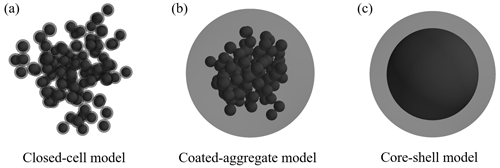Figure 1Geometries of the fractal BC aggregate model, with Ns=100, and the core–shell model. Panel (a) shows the closed-cell model with Df=2.4 and Vf=0.40, (b) the coated-aggregate model with Df=2.6 and Vf=0.10, and (c) the core–shell model with Vf=0.40.

During the aging process in the atmosphere, the surface of BC will be covered by organic and inorganic salt, forming complex coating structures (Kholghy et al., 2013). In order to more accurately simulate BC particles, two fractal aggregate models that are suitable for the actual morphology of BC are selected for numerical simulation, and as shown in Fig. 1a and b, the closed-cell and coated-aggregate models stand for thinly coated BC and thickly coated BC, respectively. The mixing state is represented by the ratio of the volume equivalent sphere diameter of a coated particle to that of BC core (${D}_{\text{p,v}}/{D}_{\text{c,v}}$). In addition, as shown in Fig. 1c, the core–shell model based on the spherical assumption and applicable for Mie scattering theory is constructed, and the mixing state can be directly expressed by the ratio ${D}_{\mathrm{p}}/{D}_{\mathrm{c}}$. For the construction of the closed-cell and coated-aggregate models, the tunable diffusion-limited aggregation (DLA) software developed by Wozniak et al. (2012) was used first to generate the bare BC fractal aggregate, then spherical coatings were added based on the original aggregate (Liu et al., 2023a). The ratio ${D}_{\text{p,v}}/{D}_{\text{c,v}}$ can be obtained from the volume fraction of the BC core (Vf) according to

$\begin{array}{}\text{(3)}& {V}_{\mathrm{f}}=\frac{{V}_{\mathrm{BC}}}{{V}_{\text{total}}},\text{(4)}& \frac{{D}_{\text{p,v}}}{{D}_{\text{c,v}}}=\frac{\mathrm{1}}{\sqrt[\mathrm{3}]{{V}_{\mathrm{f}}}},\text{(5)}& {D}_{\text{c,v}}=\mathrm{2}{a}_{\mathrm{0}}\sqrt[\mathrm{3}]{{N}_{\mathrm{s}}},\end{array}$

where VBC and Vtotal are the volume of the BC core and the whole coated BC particle, respectively.

In this study, the value of the fractal prefactor (kf) is assumed to be 1.20 (Sorensen and Roberts, 1997), and the radius of the BC monomer (a0) is fixed at 20 nm which is a typical value observed in experiments (Li et al., 2003). Furthermore, Ns ranges from 50 to 2000 with a step size of 50, which covers the Dc measured by SP2. The remaining microphysical parameters Df and Vf also affect the morphology of the fractal aggregate model, and the corresponding relationships between ${D}_{\text{p,v}}/{D}_{\text{c,v}}$ and Vf are shown in Table 1.

## 2.2 Numerical simulation of optical properties

Many methods have been used to calculate the optical properties of BC fractal aggregates, such as the Rayleigh–Debye–Gans (RDG) approximation, the discrete dipole approximation (DDA), and the T-matrix method (Adachi et al., 2010; Li et al., 2016; Mishchenko et al., 2013). As one of the most computationally efficient and accurate methods, the multiple-sphere T-matrix method (MSTM) was developed based on the T-matrix theory and employs the additional theorem of vector spherical wave functions to account for the interactions between different spherical monomers in multiple-sphere systems (Mackowski, 2014). The MSTM code has been widely used in numerical simulation studies to calculate the optical properties of BC fractal aggregates (He et al., 2015). In this study, the wavelength of the optical property simulation is set to 1064 nm, which is the same as the laser wavelength used in the SP2. Typically, the refractive indices of the BC core and coating materials were assumed to be 2.26–1.26i and 1.50 + 0i, respectively, and the densities of the BC core (ρBC) and coatings (ρcoating) were set to 1.80 and 1.20 g cm−3 (Zhang et al., 2019; Liu et al., 2014; Turpin and Lim, 2001). In addition, the selections of the refractive index and density are consistent for both MSTM calculation and Mie scattering calculation.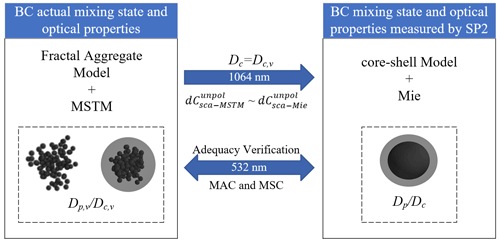Figure 2Overview of the methodology of both the BC mixing state retrieval, and the verification of optical property at 532 nm predicted based on SP2 retrieval results.

The two scattering signal detectors of the SP2 are distributed at forward scattering and backward scattering directions to simultaneously measure the forward and backward scattering of each particle, which have view angles in the (θ) ranges of 13–77 and 103–167, respectively (Wu et al., 2023). In addition, the solid angle (ΔΩ) of light collection for the detectors can be calculated according to Moteki and Kondo (2007):

$\begin{array}{}\text{(6)}& \mathrm{\Delta }\mathrm{\Omega }=\underset{\mathrm{\Delta }\mathit{\phi }}{\int }\mathrm{d}\mathit{\phi }\underset{\mathrm{\Delta }\mathit{\theta }}{\int }\mathrm{sin}\mathit{\theta }\mathrm{d}\mathit{\theta },\end{array}$

where the azimuth angle (φ) ranges from 60 to 120. Furthermore, the differential scattering cross-section of the coated soot particle is as follows:

$\begin{array}{}\text{(7)}& \frac{\mathrm{d}{C}_{\text{sca}}}{\mathrm{d}\mathrm{\Omega }}=\frac{{F}_{\mathrm{11}}}{{k}^{\mathrm{2}}},\end{array}$

where F11 is the scattering phase function and $k=\mathrm{2}\mathit{\pi }/\mathit{\lambda }$ denotes the wavenumber.

## 2.3 Retrieval of the mixing state of BC

The detected incandescent signals of SP2 can be employed to determine the mass of each single BC core, then the diameter of the BC core (Dc) can be deduced based on its density. The leading-edge-only (LEO) fit method developed by Gao et al. (2007) constructs a complete Gaussian scattering function using the scattering signal of each coated BC particle before it is perturbed by the laser, and the Gaussian scattering function can be used to determine the differential scattering cross-section of the coated BC particle. Furthermore, the diameter of coated BC particles (Dp) can be retrieved using Mie scattering theory. Based on the retrieval principle of SP2, the retrieval of the mixing state ${D}_{\mathrm{p}}/{D}_{\mathrm{c}}$ with the differential scattering cross-section of the fractal soot particle as a reference is the key of this study.

The specific retrieval process is shown in Fig. 2. First, the differential optical property of the BC fractal aggregate models with preset ${D}_{\text{p,v}}/{D}_{\text{c,v}}$ at 1064 nm are calculated based on the MSTM code. Second, the optical properties of the core–shell model are calculated using Mie scattering theory, with the value of Dc being the same as the Dc,v. Lastly, the core–shell model whose differential scattering cross-section has the smallest difference from that of fractal aggregate model is retrieved, and its ${D}_{\mathrm{p}}/{D}_{\mathrm{c}}$ is regarded as the retrieved mixing state of the coated BC particle. Furthermore, a simplified conceptual retrieval method based on ordinary least squares was defined as follows:

$\begin{array}{}\text{(8)}& {\mathit{\chi }}^{\mathrm{2}}={\left[\frac{\mathrm{d}{C}_{\text{sca-MSTM}}\left({D}_{\text{p,v}}\right)-\mathrm{d}{C}_{\text{sca-Mie}}\left({D}_{\mathrm{p}}\right)}{\mathrm{d}{C}_{\text{sca-MSTM}}\left({D}_{\text{p,v}}\right)}\right]}^{\mathrm{2}},\end{array}$

where dCsca-MSTM and dCsca-Mie are the simulated differential scattering cross-sections of BC fractal aggregate models and core–shell models, respectively. In addition, the relative error (RE) is used to represent the mixing state retrieval error of BC:

$\begin{array}{}\text{(9)}& \mathrm{RE}=\frac{{D}_{\mathrm{p}}/{D}_{\mathrm{c}}-{D}_{\text{p,v}}/{D}_{\text{c,v}}}{{D}_{\text{p,v}}/{D}_{\text{c,v}}}×\mathrm{100}\phantom{\rule{0.125em}{0ex}}\mathit{%}.\end{array}$

Figure 2 also shows the verification process when SP2 retrieval results are used to predict the optical properties of coated BC at 532 nm. The optical properties of BC fractal aggregate models with the preset particle size (Dp,v) and the core–shell model with the retrieved particle size (Dp) at 532 nm are calculated based on the MSTM code and Mie scattering theory, respectively. The calculated normalized scattering and absorption properties are provided in Sect. 3.3.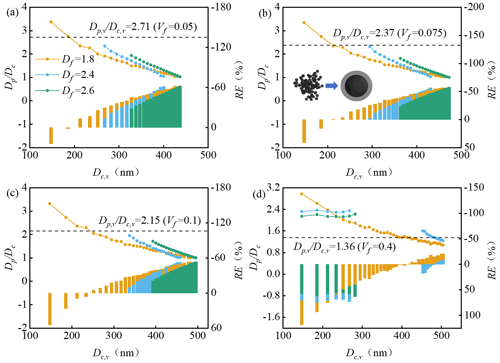Figure 3Retrieved mixing state (${D}_{\mathrm{p}}/{D}_{\mathrm{c}}$) and relative error (RE) as functions of volume equivalent diameter (Dc,v) for thinly coated BC particles with different Df and ${D}_{\text{p,v}}/{D}_{\text{c,v}}$. The colored lines stand for retrieved ${D}_{\mathrm{p}}/{D}_{\mathrm{c}}$ and the colored bars stand for RE. Panel (a) shows closed-cell models with ${D}_{\text{p,v}}/{D}_{\text{c,v}}=\mathrm{2.71}$, (b) closed-cell models with ${D}_{\text{p,v}}/{D}_{\text{c,v}}=\mathrm{2.37}$, (c) closed-cell models with ${D}_{\text{p,v}}/{D}_{\text{c,v}}=\mathrm{2.15}$, and (d) closed-cell models with ${D}_{\text{p,v}}/{D}_{\text{c,v}}=\mathrm{1.36}$.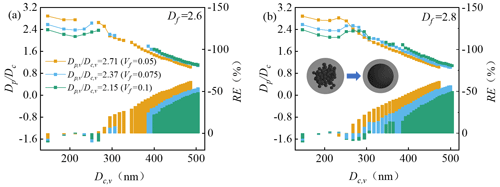Figure 4Retrieved mixing state (${D}_{\mathrm{p}}/{D}_{\mathrm{c}}$) and relative error (RE) as functions of volume equivalent diameter (Dc,v) for thickly coated BC particles with different Df and ${D}_{\text{p,v}}/{D}_{\text{c,v}}$. Panel (a)shows coated aggregates with Df=2.60 and (b) shows coated aggregates with Df=2.80. The colored lines stand for retrieved ${D}_{\mathrm{p}}/{D}_{\mathrm{c}}$ and the colored bars stand for RE.

3 Results and discussion

## 3.1 Effect of BC aerosol morphology on the retrieval of the mixing state

When the combination of forward and backward differential scattering cross-sections is employed to retrieve the BC mixing state, ${D}_{\mathrm{p}}/{D}_{\mathrm{c}}$ for a noteworthy amount of coated soot particles cannot be retrieved based on Mie scattering theory due to the different optical properties between spherical and fractal models, which means that the mixing states of some coated soot particles may be ignored. Therefore, the retrieval performances of the BC mixing state based on forward and backward scattering are further explored, separately. Comprehensive comparisons showed that the retrieval based on forward scattering can avoid the coated BC particles being missed by SP2 to the greatest extent. Therefore, the retrieved results of mixing states based on forward scattering are selected for further discussion in the following sections. Figure 3 shows the retrieval results and relative errors (RE) of mixing states of thinly coated soot under different fractal dimensions (Df) and volume equivalent particle diameter ratio shell and core (${D}_{\text{p,v}}/{D}_{\text{c,v}}$). The colored lines and bars stand for retrieved ${D}_{\mathrm{p}}/{D}_{\mathrm{c}}$ and RE, respectively. The dashed black line in each panel is the preset value of${D}_{\text{p,v}}/{D}_{\text{c,v}}$. Since the shell is larger than the core for the core–shell model, a lower limit (${D}_{\mathrm{p}}/{D}_{\mathrm{c}}=\mathrm{1}$) exists for the retrieval results. Figure S1 in the Supplement shows the variation of differential scattering cross-sections with a coated particle diameter Dp of core–shell models with a different core diameter (Dc= 252.0, 337.4, 447.5 nm), where the solid and dotted lines indicate differential scattering cross-sections integral from 13 to 77 and from 103 to 167, respectively. There are maximum and minimum values of the forward and backward differential scattering cross-sections, respectively, and there are apparent differences in the corresponding optical properties of fractal models and core–shell models, so the value of Dp for some fractal soot particles cannot be retrieved, leading to missed points in the figures of retrieved results of ${D}_{\mathrm{p}}/{D}_{\mathrm{c}}$ and RE. For the thinly coated soot with ${D}_{\text{p,v}}/{D}_{\text{c,v}}$ larger than 2.15, as shown in Fig. 3a–c, most of the relative errors of retrieved ${D}_{\mathrm{p}}/{D}_{\mathrm{c}}$ are negative, especially for soot aerosols with larger preset ${D}_{\text{p,v}}/{D}_{\text{c,v}}$ and fractal dimension, which indicates that SP2 underestimates ${D}_{\mathrm{p}}/{D}_{\mathrm{c}}$ of these coated soot particles. The retrieval results of ${D}_{\mathrm{p}}/{D}_{\mathrm{c}}$ at different fractal dimensions have similar variation trends with the increase in the volume equivalent diameter of soot core. The ${D}_{\mathrm{p}}/{D}_{\mathrm{c}}$ of all the preset ${D}_{\text{p,v}}/{D}_{\text{c,v}}$ can be retrieved for the closed-cell model with Df=1.8. However, fewer results can be retrieved as fractal dimension increases; thus, the retrieved ${D}_{\mathrm{p}}/{D}_{\mathrm{c}}$ of SP2 may lose data of large amounts of slightly aged and compact atmospheric BC. In addition, as the fractal dimension decreases, the retrieved results become smaller while the absolute values of the relative error become larger. It should be noted that some different phenomenon appears in the retrieved mixing state when the preset ${D}_{\text{p,v}}/{D}_{\text{c,v}}$ is small and the fractal dimension is large. As can be seen from Fig. 3d, the variations in retrieval results for closed-cell models with Df= 2.40 and 2.60 is different from that of Fig. 3a–c, and ${D}_{\mathrm{p}}/{D}_{\mathrm{c}}$ can be well retrieved at small Dc,v. In addition, Fig. S2 demonstrates the retrieval results for ${D}_{\text{p,v}}/{D}_{\text{c,v}}=\mathrm{1.13}$, similarly, ${D}_{\mathrm{p}}/{D}_{\mathrm{c}}$ can be retrieved at small Dc,v for closed-cell models with Df=2.40 and 2.60. Figure 4 shows the retrieval results and relative errors (RE) of mixing states of thickly coated particles. It can be seen that although the retrieval results for thickly coated BC particles also have missed points, the overall trend is clear and consistent at different particle fractal dimensions, and the largest RE of coated particles is about 62 %. With the increase in particle size, the retrieval results of ${D}_{\mathrm{p}}/{D}_{\mathrm{c}}$ also gradually converge to 1. Overall, the relative error of ${D}_{\mathrm{p}}/{D}_{\mathrm{c}}$ for thickly coated BC particles is smaller than that for thinly coated BC particles, and the retrieval results for thickly coated BC particles cover a wider range of particle sizes, which suggests that SP2 is more accurate in measuring the mixing state of thickly coated BC particles than that of thinly coated BC particles.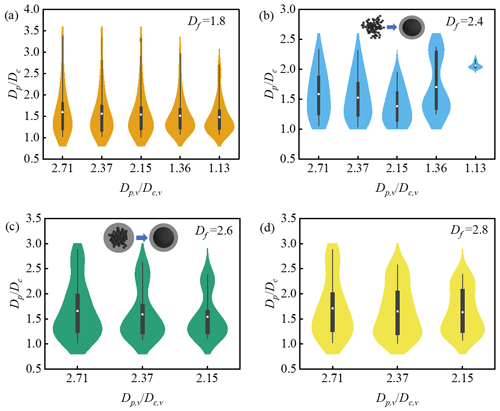Figure 5The distributions of retrieved ${D}_{\mathrm{p}}/{D}_{\mathrm{c}}$ for different models with different fractal dimensions. Panel (a) shows thinly coated BC with Df=1.80, (b) thinly coated BC with Df=2.40, (c) thickly coated BC with Df=2.60, and (d) thickly coated BC with Df=2.80. The black box represents the 25th and 75th percentiles, the whisker represents the maximum and minimum values of the retrieved results, and the white dot in the boxes represents the average value of retrieved ${D}_{\mathrm{p}}/{D}_{\mathrm{c}}$.

The distribution of retrieved results of mixing states for single particles with different fractal dimensions over the entire particle size range is shown in Fig. 5, and the filling width represents the probability distribution of retrieved ${D}_{\mathrm{p}}/{D}_{\mathrm{c}}$. As mentioned above, when ${D}_{\text{p,v}}/{D}_{\text{c,v}}$ decreases and Df increases, ${D}_{\mathrm{p}}/{D}_{\mathrm{c}}$ of some coated soot particles cannot be retrieved, which is why the data point for ${D}_{\text{p,v}}/{D}_{\text{c,v}}=\mathrm{1.13}$ and 1.36, is smaller than the other three points, especially for ${D}_{\text{p,v}}/{D}_{\text{c,v}}=\mathrm{1.13}$. Therefore, the particle mixing states with a large amount of missed retrieval results are not considered in both the prediction adequacy verification of optical properties and the radiative forcing of aged soot aerosols in Sects. 3.3 and 3.4. In general, the mean value of retrieved ${D}_{\mathrm{p}}/{D}_{\mathrm{c}}$ decreases as the preset ${D}_{\text{p,v}}/{D}_{\text{c,v}}$ decreases. It can be seen from the figures that the probability distribution of retrieved mixing states are similar for particles employing the same model. The ${D}_{\mathrm{p}}/{D}_{\mathrm{c}}$ for soot with more compact structures has a higher retrieval accuracy and the distributions are more uniform. As for the comparison of these two morphological models for soot with different aging states, the retrieved ${D}_{\mathrm{p}}/{D}_{\mathrm{c}}$ of the coated-aggregate model is closer to the preset values, which confirms that the mixing state retrieval error of SP2 is relatively smaller for thickly coated BC particles.

## 3.2 Retrieved mixing state for particles with a certain size distribution

It is known from previous observations and experiments that the bulk optical properties of realistic atmospheric BC particles are integrated over a certain particle size distribution. In order to better understand the accuracy of SP2 on the ${D}_{\mathrm{p}}/{D}_{\mathrm{c}}$ retrieval results of the bulk BC particles, the retrieved results for particle groups are also considered in this study. It is assumed that the equivalent volume diameters of coated BC particles follow the log-normal distribution

$\begin{array}{}\text{(10)}& n\left(d\right)=\frac{\mathrm{1}}{\sqrt{\mathrm{2}\mathit{\pi }}d\mathrm{ln}\left({\mathit{\sigma }}_{\mathrm{g}}\right)}\mathrm{exp}\left[-{\left(\frac{\mathrm{ln}\left(d\right)-\mathrm{ln}\left({d}_{\mathrm{g}}\right)}{\sqrt{\mathrm{2}}\mathrm{ln}\left({\mathit{\sigma }}_{\mathrm{g}}\right)}\right)}^{\mathrm{2}}\right],\end{array}$

where σg is the geometric standard deviation, dg is the geometric mean diameter, and d is the diameter of the sphere with the same volume as that of the whole coated BC aggregates (i.e., volume equivalent sphere). Particle size distribution of coated BC aggregates with dg=0.15µm (Yu and Luo, 2009) and σg=1.59 (Zhang et al., 2012) is considered, and these BC particles have the same preset ${D}_{\mathrm{p}}/{D}_{\mathrm{c}}$. The retrieved ${D}_{\mathrm{p}}/{D}_{\mathrm{c}}$ and relative errors of the BC particles with the size distribution mentioned above are shown in Table 2. For the thinly coated BC particles with Df=1.80, retrieval results decrease with the increase in preset ${D}_{\text{p,v}}/{D}_{\text{c,v}}$, but there is not obvious regularity due to the missed retrieval results of the closed-cell models with Df=2.40 and 2.60. In addition, the relative errors for closed-cell particle groups are larger than those of coated-aggregate particle groups.

Table 2Retrieved ${D}_{\mathrm{p}}/{D}_{\mathrm{c}}$ and relative errors of BC particle groups.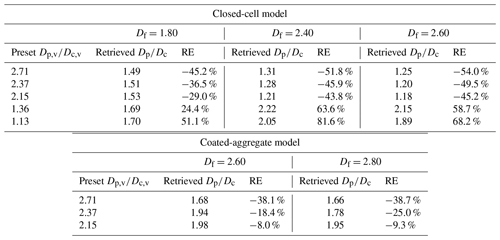## 3.3 Verification of adequacy when SP2 retrieval results are employed to predict optical properties of BC

The optical equivalent diameter Dp and mixing state (${D}_{\mathrm{p}}/{D}_{\mathrm{c}}$) can be retrieved by SP2 based on Mie scattering. Then, all the optical properties of black carbon particles or even optical properties at other wavelengths can be predicted based on Mie scattering calculation of the core–shell model. In this study, the prediction accuracy of optical properties at 532 nm are selected as a typical example. The optical simulation results of the fractal particle models are used to represent the optical properties of the actual atmospheric BC particles, while the optical properties of the core–shell model represent the predicted optical properties of the BC particles inferred from the SP2 retrieval results.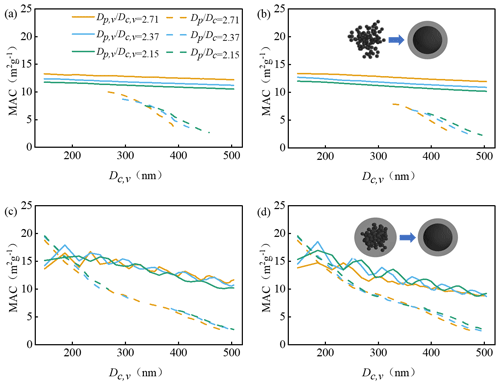Figure 6Differences in mass absorption cross-section (MAC) between the fractal particle model (solid lines) and core–shell model (dashed lines) at 532 nm. Panel (a) shows the closed-cell model with Df=2.40, (b) closed-cell model with Df=2.60, (c) coated-aggregate model with Df=2.60, and (d) coated-aggregate model with Df=2.80.

The radiative forcing of BC aerosol is closely related to the absorption and scattering properties. In order to investigate the effect of retrieval errors of SP2 caused by morphological model selection on the estimation of BC radiation effect, optical properties mass scattering cross-section (MSC) and mass absorption cross-section (MAC) are calculated for further investigation:

$\begin{array}{}\text{(11)}& \mathrm{MAC}=\frac{{C}_{\text{sca}}}{{m}_{\mathrm{BC}}},\text{(12)}& \mathrm{MSC}=\frac{{C}_{\text{abs}}}{{m}_{\text{total}}},\text{(13)}& {m}_{\mathrm{BC}}={\mathit{\rho }}_{\mathrm{BC}}{V}_{\mathrm{BC}},\text{(14)}& {m}_{\text{total}}={\mathit{\rho }}_{\mathrm{BC}}{V}_{\mathrm{BC}}+{\mathit{\rho }}_{\text{coating}}{V}_{\text{coating}},\text{(15)}& {V}_{\text{coating}}=\left(\mathrm{1}-{V}_{\mathrm{f}}\right){V}_{\mathrm{BC}}/{V}_{\mathrm{f}},\end{array}$

where mBC and mtotal are the masses of the BC core and the whole coated BC particle, and Vcoating is the volume of coating material. The values of density of both BC and coating are mentioned in Sect. 2.2.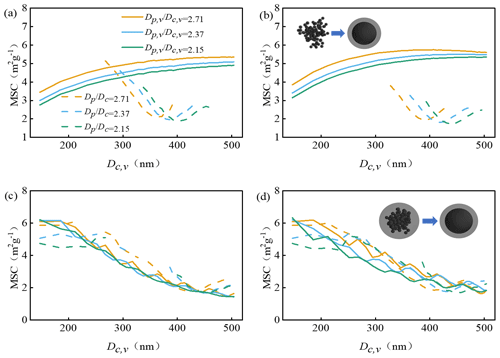Figure 7Similar to Fig. 6, but the solid and dashed lines represent the mass scattering cross-section (MSC) of fractal particle model and core–shell model, respectively.

Figures 6 and 7 demonstrate the mass absorption cross-section and mass scattering cross-section of fractal models and core–shell models, respectively. The mass absorption cross-section of the closed-cell model slightly increases with the increase in both ${D}_{\text{p,v}}/{D}_{\text{c,v}}$ and Df, but the size of BC core has little influence. The calculation results of the optical equivalent core–shell model based on SP2 retrieval results decrease with the increase in BC core size, and decrease with the increase in ${D}_{\text{p,v}}/{D}_{\text{c,v}}$ for large particles. Furthermore, the predicted MAC of coated particles is smaller than the actual MAC when the size of the BC core is larger than about 260 nm. As shown in Fig. 6c and d, the fractal dimension and mixing state have small effects on the mass absorption cross-section of the coated-aggregate model, but the differences in MAC between the coated-aggregate model and the core–shell model is apparent. This indicates that the predicted results of the mass absorption cross-section deviate significantly from the actual values of the thickly coated BC particles, and the maximum relative error is up to about 78 %.

Figure 7 demonstrates that the mass scattering cross-sections of the closed-cell model increase with particle size, fractal dimension, and mixing state, and they are obviously larger than the corresponding results of the core–shell model in most cases over the entire particle size range, indicating that the predicted results of the MSC based on SP2 retrieval results underestimate the mass scattering cross-sections of coated BC at 532 nm wavelength. In contrast, the mass scattering cross-sections of both the coated-aggregate model and the core–shell model decrease with the increase in particle size, as shown in Fig. 7c and d, but the prediction error of the MSC is less of a regularity and hard to parameterize.

## 3.4 Effects of SP2 retrieval errors on the estimation of BC radiative forcing

The microphysical properties of black carbon (morphological structure, size distribution, and mixing state) have significant impacts on their optical properties, which further affect the radiative effect of black carbon. Lu et al. (2020) revealed that the uncertainty of the radiative forcing and heating rate of BC due to different geometries and size distributions of BC is less than 30 %, while the uncertainty due to the mixing state of BC is as high as 80 %. The SP2 retrieves the mixing state of BC based on the Mie scattering theory and BC particles are assumed to have spherical core–shell structures. But the core–shell model is seriously out of line with the actual morphology of BC, so the retrieved mixing state has unavoidable errors, which in turn affect the estimation of the radiative effect of BC. In this study, the simple forcing efficiency (SFE) equation developed by Bond and Bergstrom (2006) is employed to quantify the radiative forcing evaluation errors caused by the mixing state retrieval errors of SP2. The SFE is defined as radiative forcing normalized by BC mass and represents the energy added to the earth's atmospheric system by a given mass of particles in the atmosphere:

$\begin{array}{}\text{(16)}& \begin{array}{rl}\frac{\mathrm{d}\mathrm{SFE}}{\mathrm{d}\mathit{\lambda }}=& \phantom{\rule{0.25em}{0ex}}-\frac{\mathrm{1}}{\mathrm{4}}\frac{\mathrm{d}S\left(\mathit{\lambda }\right)}{\mathrm{d}\mathit{\lambda }}{\mathit{\tau }}^{\mathrm{2}}\left(\mathrm{1}-{F}_{\mathrm{c}}\right)\\ & ×\left[\mathrm{2}\left(\mathrm{1}-{a}_{\mathrm{s}}{\right)}^{\mathrm{2}}\mathit{\beta }\left(\mathit{\lambda }\right)×\mathrm{MSC}\left(\mathit{\lambda }\right)-\mathrm{4}{a}_{\mathrm{s}}\mathrm{MAC}\left(\mathit{\lambda }\right)\right],\end{array}\end{array}$

where $\frac{\mathrm{d}S\left(\mathit{\lambda }\right)}{\mathrm{d}\mathit{\lambda }}$ is the spectral solar irradiance according to ASTM G173-03, the atmospheric transmittance τ=0.79, the cloud fraction Fc=0.6, typical urban surface albedo as=0.19, and the backscatter fraction β=0.15.

Tables 3 and 4 show the calculated results of SFE at 532 and 1064 nm, and it can be found that all the values of radiative forcing of coated BC particles are positive. The “actual value” is the SFE of actual thinly and thickly coated BC particles represented by the closed-cell model and coated-aggregate model, and the optical properties are obtained using MSTM. The “retrieved value” is the SFE obtained using recalculated MSC and MAC based on the optical equivalent core–shell models from SP2 retrieval results. The effects of the SP2 retrieval error on SFE are disparate at different wavelengths, and the relative errors for all the considered particles in this study range from 55.4 % to 0.2 % at 1064 nm and 94.5 % to 69.2 % at 532 nm. The distinctions of SFE between the closed-cell model and the corresponding optical equivalent core–shell model are more significant than the distinctions of SFE between the coated-aggregate model and the core–shell model at 1064 nm, which indicates that the SP2 retrieval error has more significant influence on the radiative forcing of the thinly coated BC. In summary, the effects of the SP2 retrieval errors on the estimation of the radiative forcing of coated BC is noteworthy and cannot be ignored.

Table 3The SFE values of both the core–shell models used to interpret the SP2 measurements and the fractal soot models at 1064 nm.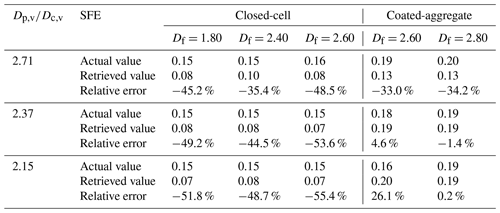Table 4The SFE values of both the core–shell models used to interpret the SP2 measurements and the fractal soot models at 1064 nm.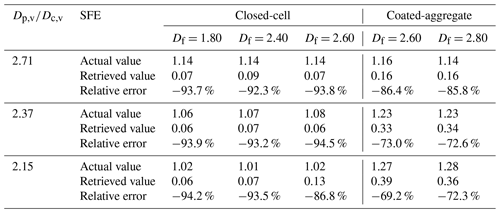4 Conclusions

BC aerosols have strong light absorption capacity compared with other atmospheric aerosols, and they can disturb the regional and global radiation balance and produce positive radiative forcing by absorbing solar radiation, which eventually leads to global warming. The content and structure of coating significantly change the mixing state (${D}_{\mathrm{p}}/{D}_{\mathrm{c}}$) of black carbon particles, and the lens effect caused by coating has important and complicated effects on the optical properties of black carbon, which brings great uncertainty to the radiative effect and ultimately affects the accurate assessment of the climate effect of black carbon aerosols.

Single-particle soot photometer (SP2) employs the spherical core–shell model to represent coated BC particles, but this inappropriate model introduces errors into the ${D}_{\mathrm{p}}/{D}_{\mathrm{c}}$ retrieval results and is not conducive to accurately obtaining the mixing state of coated fractal BC particles in the atmosphere. This study quantifies the SP2 retrieval error of the mixing state of coated BC particles using typical fractal morphological models of both thinly and thickly coated BC from the perspective of numerical simulations and evaluates the effect of retrieval error on the estimation of radiative forcing of BC aerosols. The main conclusions are summarized as follows:

1. The mixing state of most BC particles retrieved by SP2 based on Mie scattering is underestimated compared with the realistic ${D}_{\mathrm{p}}/{D}_{\mathrm{c}}$. Relative error in the retrieved mixing state of single BC particles under different coating conditions is significantly different. The relative error of thinly coated BC particles represented by the closed-cell model is larger than that of thickly coated BC particles represented by the coated-aggregate model. With the decrease of fractal dimension, the relative errors of the mixing state of thinly coated BC particles with loose structures increase.

2. For BC groups whose particle size follows a certain log-normal distribution, the SP2 retrieval results of ${D}_{\mathrm{p}}/{D}_{\mathrm{c}}$ will be underestimated. The relative errors of ${D}_{\mathrm{p}}/{D}_{\mathrm{c}}$ for the closed-cell model are larger than that for the coated-aggregate model, indicating that the SP2 retrieval accuracy of the mixing state of thickly coated BC particles is better than that of thinly coated BC particles.

3. When the ${D}_{\mathrm{p}}/{D}_{\mathrm{c}}$ retrieved by SP2 are used to predict optical properties of coated BC, it can be found that the predicted MAC and MSC at 532 nm recalculated based on the core–shell model have significant discrepancies with regard to those of realistic thinly and thickly coated BC particles. The largest difference between the predicted MAC and the reference value is up to about 78 %.

4. The retrieval errors of mixing states have larger effects on the estimation accuracy of radiative forcing for thinly coated BC particles than that for thickly coated BC particles at both 1064 and 532 nm wavelengths. Furthermore, the relative error of estimated radiative forcing of thinly coated BC particles reaches about 55 % at 1064 nm, while it reaches about 95 % at 532 nm.

This study is a pilot work for the characterization of possible retrieval errors in mixing states of coated soot aerosols using SP2 and Mie theory. For future work, more morphological models that are suitable for modeling the microstructure of coated soot aerosols should be considered, the retrieval performance of mixing state based on forward scattering, backward scattering, and their combination should be explored, and unified parameterization schemes of retrieval errors are much needed. Furthermore, comprehensive field or laboratory studies for the validation of the possible errors in mixing states are also future directions worth the effort.

Code and data availability

Processed data for this study are available online (https://doi.org/10.13140/RG.2.2.29017.90729, Liu et al., 2023b). The multi-sphere T-matrix code used in this study is available online (https://github.com/oldoldstone/MSTM, Mishchenko and Mackowski, 2023).

Supplement

Author contributions

JL: conceptualization, methodology, funding acquisition, and co-writing of the original draft; GW: methodology, experiment, formal analysis, and co-writing of the original draft; CZ: experiment, data curation, and manuscript review and editing; DZ: formal analysis, data curation, and manuscript review and editing; and LW: data curation as well as manuscript review and editing.

Competing interests

The contact author has declared that none of the authors has any competing interests.

Disclaimer

Publisher’s note: Copernicus Publications remains neutral with regard to jurisdictional claims made in the text, published maps, institutional affiliations, or any other geographical representation in this paper. While Copernicus Publications makes every effort to include appropriate place names, the final responsibility lies with the authors.

Acknowledgements

We particularly thank Michael I. Mishchenko and Daniel W. Mackowski for the MSTM code. We also appreciate the support of the supercomputing center of Hebei University.

Financial support

This research has been supported by the National Natural Science Foundation of China (grant no. 42305082), the Natural Science Foundation of Hebei Province (grant no. D2021201002), and the High-level Talents Research Start Project of Hebei University (grant no. 521000981417).

Review statement

This paper was edited by Meng Gao and reviewed by Joshua Schwarz and two anonymous referees.

References

Adachi, K., Chung, S. H., and Buseck, P. R.: Shapes of soot aerosol particles and implications for their effects on climate, J. Geophys. Res.-Atmos., 115, 9, https://doi.org/10.1029/2009jd012868, 2010.

Bond, T. C. and Bergstrom, R. W.: Light absorption by carbonaceous particles: An investigative review, Aerosol Sci. Tech., 40, 27–67, https://doi.org/10.1080/02786820500421521, 2006.

China, S., Mazzoleni, C., Gorkowski, K., Aiken, A. C., and Dubey, M. K.: Morphology and mixing state of individual freshly emitted wildfire carbonaceous particles, Nat. Commun., 4, 2122, https://doi.org/10.1038/ncomms3122, 2013.

China, S., Scarnato, B., Owen, R. C., Zhang, B., Ampadu, M. T., Kumar, S., Dzepina, K., Dziobak, M. P., Fialho, P., Perlinger, J. A., Hueber, J., Helmig, D., Mazzoleni, L. R., and Mazzoleni, C.: Morphology and mixing state of aged soot particles at a remote marine free troposphere site: Implications for optical properties, Geophys. Res. Lett., 42, 1243–1250, https://doi.org/10.1002/2014gl062404, 2015.

Ching, J., Riemer, N., and West, M.: Black carbon mixing state impacts on cloud microphysical properties: Effects of aerosol plume and environmental conditions, J. Geophys. Res.-Atmos., 121, 5990–6013, https://doi.org/10.1002/2016jd024851, 2016.

Forestieri, S. D., Helgestad, T. M., Lambe, A. T., Renbaum-Wolff, L., Lack, D. A., Massoli, P., Cross, E. S., Dubey, M. K., Mazzoleni, C., Olfert, J. S., Sedlacek III, A. J., Freedman, A., Davidovits, P., Onasch, T. B., and Cappa, C. D.: Measurement and modeling of the multiwavelength optical properties of uncoated flame-generated soot, Atmos. Chem. Phys., 18, 12141–12159, https://doi.org/10.5194/acp-18-12141-2018, 2018.

Gao, R. S., Schwarz, J. P., Kelly, K. K., Fahey, D. W., Watts, L. A., Thompson, T. L., Spackman, J. R., Slowik, J. G., Cross, E. S., Han, J. H., Davidovits, P., Onasch, T. B., and Worsnop, D. R.: A novel method for estimating light-scattering properties of soot aerosols using a modified single-particle soot photometer, Aerosol Sci. Tech., 41, 125–135, https://doi.org/10.1080/02786820601118398, 2007.

He, C., Liou, K.-N., Takano, Y., Zhang, R., Levy Zamora, M., Yang, P., Li, Q., and Leung, L. R.: Variation of the radiative properties during black carbon aging: theoretical and experimental intercomparison, Atmos. Chem. Phys., 15, 11967–11980, https://doi.org/10.5194/acp-15-11967-2015, 2015.

Huang, X., Wang, Z., and Ding, A.: Impact of Aerosol-PBL Interaction on Haze Pollution: Multiyear Observational Evidences in North China, Geophys. Res. Lett., 45, 8596–8603, https://doi.org/10.1029/2018gl079239, 2018.

Jacobson, M. Z.: Climate response of fossil fuel and biofuel soot, accounting for soot's feedback to snow and sea ice albedo and emissivity, J. Geophys. Res.-Atmos., 109, D21201, https://doi.org/10.1029/2004jd004945, 2004.

Kanngiesser, F. and Kahnert, M.: Calculation of optical properties of light-absorbing carbon with weakly absorbing coating: A model with tunable transition from film-coating to spherical-shell coating, J. Quant. Spectrosc. Ra., 216, 17–36, https://doi.org/10.1016/j.jqsrt.2018.05.014, 2018.

Kholghy, M., Saffaripour, M., Yip, C., and Thomson, M. J.: The evolution of soot morphology in a laminar coflow diffusion flame of a surrogate for Jet A-1, Combust. Flame, 160, 2119–2130, https://doi.org/10.1016/j.combustflame.2013.04.008, 2013.

Kompalli, S. K., Babu, S. N. S., Moorthy, K. K., Satheesh, S. K., Gogoi, M. M., Nair, V. S., Jayachandran, V. N., Liu, D., Flynn, M. J., and Coe, H.: Mixing state of refractory black carbon aerosol in the South Asian outflow over the northern Indian Ocean during winter, Atmos. Chem. Phys., 21, 9173–9199, https://doi.org/10.5194/acp-21-9173-2021, 2021.

Laborde, M., Schnaiter, M., Linke, C., Saathoff, H., Naumann, K.-H., Möhler, O., Berlenz, S., Wagner, U., Taylor, J. W., Liu, D., Flynn, M., Allan, J. D., Coe, H., Heimerl, K., Dahlkötter, F., Weinzierl, B., Wollny, A. G., Zanatta, M., Cozic, J., Laj, P., Hitzenberger, R., Schwarz, J. P., and Gysel, M.: Single Particle Soot Photometer intercomparison at the AIDA chamber, Atmos. Meas. Tech., 5, 3077–3097, https://doi.org/10.5194/amt-5-3077-2012, 2012.

Li, J., Posfai, M., Hobbs, P. V., and Buseck, P. R.: Individual aerosol particles from biomass burning in southern Africa: 2, Compositions and aging of inorganic particles, J. Geophys. Res.-Atmos., 108, 12, https://doi.org/10.1029/2002jd002310, 2003.

Li, J., Liu, C., Yin, Y., and Kumar, K. R.: Numerical investigation on the angstrom ngstrom exponent of black carbon aerosol, J. Geophys. Res.-Atmos., 121, 3506–3518, https://doi.org/10.1002/2015jd024718, 2016.

Liu, D., Allan, J. D., Young, D. E., Coe, H., Beddows, D., Fleming, Z. L., Flynn, M. J., Gallagher, M. W., Harrison, R. M., Lee, J., Prevot, A. S. H., Taylor, J. W., Yin, J., Williams, P. I., and Zotter, P.: Size distribution, mixing state and source apportionment of black carbon aerosol in London during wintertime, Atmos. Chem. Phys., 14, 10061–10084, https://doi.org/10.5194/acp-14-10061-2014, 2014.

Liu, H., Pan, X., Wang, D., Liu, X., Tian, Y., Yao, W., Lei, S., Zhang, Y., Li, J., Lei, L., Xie, C., Fu, P., Sun, Y., and Wang, Z.: Mixing characteristics of black carbon aerosols in a coastal city using the CPMA-SP2 system, Atmos. Res., 265, https://doi.org/10.1016/j.atmosres.2021.105867, 2022.

Liu, J., Wang, L., Wang, G. Y., and Zhang, X. H.: Numerical investigation on the accuracy of size information of fractal soot aerosols retrieved by lidar: Optical property, morphology effect, and parameterization scheme, J. Quant. Spectrosc. Ra., 295, 12, https://doi.org/10.1016/j.jqsrt.2022.108435, 2023a.

Liu, J., Wang, G. Y., Zhu, C. C., Zhou, D. H., and Wang, L.: DpDc retrieval error of BC using SP2, ResearchGate [data set]​​​​​​​, https://doi.org/10.13140/RG.2.2.29017.90729, 2023b.

Lu, Q., Liu, C., Zhao, D. L., Zeng, C., Li, J., Lu, C. S., Wang, J. D., and Zhu, B.: Atmospheric heating rate due to black carbon aerosols: Uncertainties and impact factors, Atmos. Res., 240, 9, https://doi.org/10.1016/j.atmosres.2020.104891, 2020.

Mackowski, D. W.: A general superposition solution for electromagnetic scattering by multiple spherical domains of optically active media, J. Quant. Spectrosc. Ra., 133, 264–270, https://doi.org/10.1016/j.jqsrt.2013.08.012, 2014.

Mishchenko, M. I. and Mackowski, D. W.: The multiple-sphere T-matrix code, GitHub [code], https://github.com/oldoldstone/MSTM (last access: 27 October 2023), 2023.

Mishchenko, M. I., Liu, L., and Mackowski, D. W.: T-matrix modeling of linear depolarization by morphologically complex soot and soot-containing aerosols, J. Quant. Spectrosc. Ra., 123, 135–144, https://doi.org/10.1016/j.jqsrt.2012.11.012, 2013.

Moteki, N. and Kondo, Y.: Effects of mixing state on black carbon measurements by laser-induced incandescence, Aerosol Sci. Tech., 41, 398–417, https://doi.org/10.1080/02786820701199728, 2007.

Moteki, N. and Kondo, Y.: Method to measure time-dependent scattering cross sections of particles evaporating in a laser beam, J. Aerosol Sci., 39, 348–364, https://doi.org/10.1016/j.jaerosci.2007.12.002, 2008.

Moteki, N. and Kondo, Y.: Dependence of Laser-Induced Incandescence on Physical Properties of Black Carbon Aerosols: Measurements and Theoretical Interpretation, Aerosol Sci. Tech., 44, 663–675, https://doi.org/10.1080/02786826.2010.484450, 2010.

Moteki, N., Kondo, Y., and Adachi, K.: Identification by single-particle soot photometer of black carbon particles attached to other particles: Laboratory experiments and ground observations in Tokyo, J. Geophys. Res.-Atmos., 119, 1031–1043, https://doi.org/10.1002/2013jd020655, 2014.

Romshoo, B., Pöhlker, M., Wiedensohler, A., Pfeifer, S., Saturno, J., Nowak, A., Ciupek, K., Quincey, P., Vasilatou, K., Ess, M. N., Gini, M., Eleftheriadis, K., Robins, C., Gaie-Levrel, F., and Müller, T.: Importance of size representation and morphology in modelling optical properties of black carbon: comparison between laboratory measurements and model simulations, Atmos. Meas. Tech., 15, 6965–6989, https://doi.org/10.5194/amt-15-6965-2022, 2022.

Scarnato, B. V., Vahidinia, S., Richard, D. T., and Kirchstetter, T. W.: Effects of internal mixing and aggregate morphology on optical properties of black carbon using a discrete dipole approximation model, Atmos. Chem. Phys., 13, 5089–5101, https://doi.org/10.5194/acp-13-5089-2013, 2013.

Schwarz, J. P., Gao, R. S., Fahey, D. W., Thomson, D. S., Watts, L. A., Wilson, J. C., Reeves, J. M., Darbeheshti, M., Baumgardner, D. G., Kok, G. L., Chung, S. H., Schulz, M., Hendricks, J., Lauer, A., Karcher, B., Slowik, J. G., Rosenlof, K. H., Thompson, T. L., Langford, A. O., Loewenstein, M., and Aikin, K. C.: Single-particle measurements of midlatitude black carbon and light-scattering aerosols from the boundary layer to the lower stratosphere, J. Geophys. Res.-Atmos., 111, 15, https://doi.org/10.1029/2006jd007076, 2006.

Schwarz, J. P., Spackman, J. R., Fahey, D. W., Gao, R. S., Lohmann, U., Stier, P., Watts, L. A., Thomson, D. S., Lack, D. A., Pfister, L., Mahoney, M. J., Baumgardner, D., Wilson, J. C., and Reeves, J. M.: Coatings and their enhancement of black carbon light absorption in the tropical atmosphere, J. Geophys. Res.-Atmos., 113, 10, https://doi.org/10.1029/2007jd009042, 2008.

Schwarz, J. P., Perring, A. E., Markovic, M. Z., Gao, R. S., Ohata, S., Langridge, J., Law, D., McLaughlin, R., and Fahey, D. W.: Technique and theoretical approach for quantifying the hygroscopicity of black-carbon-containing aerosol using a single particle soot photometer, J. Aerosol Sci., 81, 110–126, https://doi.org/10.1016/j.jaerosci.2014.11.009, 2015.

Sorensen, C. M.: Light Scattering by Fractal Aggregates: A Review, Aerosol Sci. Tech., 35, 648–687, https://doi.org/10.1080/02786820117868, 2001.

Sorensen, C. M. and Roberts, G. C.: The Prefactor of Fractal Aggregates, J. Colloid Interf. Sci., 186, 447–452, https://doi.org/10.1006/jcis.1996.4664, 1997.

Turpin, B. J. and Lim, H.-J.: Species Contributions to PM2.5 Mass Concentrations: Revisiting Common Assumptions for Estimating Organic Mass, Aerosol Sci. Tech., 35, 602–610, https://doi.org/10.1080/02786820119445, 2001.

Wozniak, M., Onofri, F. R. A., Barbosa, S., Yon, J., and Mroczka, J.: Comparison of methods to derive morphological parameters of multi-fractal samples of particle aggregates from TEM images, J. Aerosol Sci., 47, 12–26, https://doi.org/10.1016/j.jaerosci.2011.12.008, 2012.

Wu, Y., Cheng, T. H., Gu, X. F., Zheng, L. J., Chen, H., and Xu, H.: The single scattering properties of soot aggregates with concentric core-shell spherical monomers, J. Quant. Spectrosc. Ra., 135, 9–19, https://doi.org/10.1016/j.jqsrt.2013.11.009, 2014.

Wu, Y., Cheng, T. H., Liu, D. T., Allan, J. D., Zheng, L. J., and Chen, H.: Light Absorption Enhancement of Black Carbon Aerosol Constrained by Particle Morphology, Environ. Sci. Technol., 52, 6912–6919, https://doi.org/10.1021/acs.est.8b00636, 2018.

Wu, Y., Cheng, T. H., Zheng, L. J., Zhang, Y. G., and Zhang, L. L.: Particle size amplification of black carbon by scattering measurement due to morphology diversity, Environ. Res. Lett., 18, 10, https://doi.org/10.1088/1748-9326/acaede, 2023.

Yu, F. and Luo, G.: Simulation of particle size distribution with a global aerosol model: contribution of nucleation to aerosol and CCN number concentrations, Atmos. Chem. Phys., 9, 7691–7710, https://doi.org/10.5194/acp-9-7691-2009, 2009.

Zeng, C., Liu, C., Li, J. N., Zhu, B., Yin, Y., and Wang, Y.: Optical Properties and Radiative Forcing of Aged BC due to Hygroscopic Growth: Effects of the Aggregate Structure, J. Geophys. Res.-Atmos., 124, 4620–4633, https://doi.org/10.1029/2018jd029809, 2019.

Zhang, K., O'Donnell, D., Kazil, J., Stier, P., Kinne, S., Lohmann, U., Ferrachat, S., Croft, B., Quaas, J., Wan, H., Rast, S., and Feichter, J.: The global aerosol-climate model ECHAM-HAM, version 2: sensitivity to improvements in process representations, Atmos. Chem. Phys., 12, 8911–8949, https://doi.org/10.5194/acp-12-8911-2012, 2012.

Zhang, X., Mao, M., Chen, H., and Tang, S.: The single scattering albedo Angstrom exponent of black carbon with brown coatings, J. Quant. Spectrosc. Ra., 259, https://doi.org/10.1016/j.jqsrt.2020.107429, 2021.

Zhang, Y., Li, M., Cheng, Y., Geng, G., Hong, C., Li, H., Li, X., Tong, D., Wu, N., Zhang, X., Zheng, B., Zheng, Y., Bo, Y., Su, H., and Zhang, Q.: Modeling the aging process of black carbon during atmospheric transport using a new approach: a case study in Beijing, Atmos. Chem. Phys., 19, 9663–9680, https://doi.org/10.5194/acp-19-9663-2019, 2019.

Zhang, Y. X., Zhang, Q., Yao, Z. L., and Li, H. Y.: Particle Size and Mixing State of Freshly Emitted Black Carbon from Different Combustion Sources in China, Environ. Sci. Technol., 54, 7766–7774, https://doi.org/10.1021/acs.est.9b07373, 2020.

Zhao, G., Tan, T., Zhu, Y., Hu, M., and Zhao, C.: Method to quantify black carbon aerosol light absorption enhancement with a mixing state index, Atmos. Chem. Phys., 21, 18055–18063, https://doi.org/10.5194/acp-21-18055-2021, 2021.

Zhao, G., Tan, T., Hu, S., Du, Z., Shang, D., Wu, Z., Guo, S., Zheng, J., Zhu, W., Li, M., Zeng, L., and Hu, M.: Mixing state of black carbon at different atmospheres in north and southwest China, Atmos. Chem. Phys., 22, 10861–10873, https://doi.org/10.5194/acp-22-10861-2022, 2022.

Zhuang, B., Liu, Q., Wang, T., Yin, C., Li, S., Xie, M., Jiang, F., and Mao, H.: Investigation on semi-direct and indirect climate effects of fossil fuel black carbon aerosol over China, Theor. Appl. Climatol., 114, 651–672, https://doi.org/10.1007/s00704-013-0862-8, 2013.